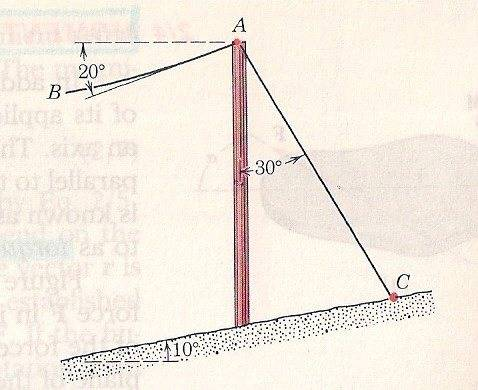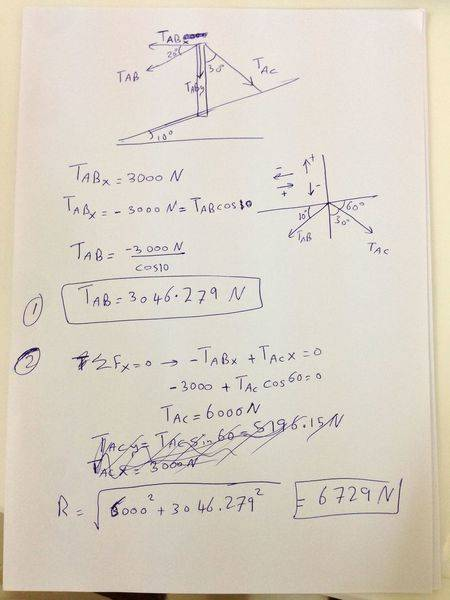# Simple Static Problem

Sam Fred

## Homework Statement

Q) A transmission cable AB exerts a 3000N force on the pole in the direction of the tangent to the cable at A (see Fig. 1). Determine the required tension T in the wire AB if the resultant of the two forces at A is to be vertical (1 point). Find the magnitude R of the resultant (2 points).ƩF = 0

## The Attempt at a SolutionI want to understand what does it mean that the 3000 N force on the pole in the direction of the tangent cable at A.

Last edited:

Homework Helper
Hi Sam Fred!I want to understand what does it mean that the 3000 N force on the pole in the direction of the tangent cable at A.

I found that very puzzling until I looked at the diagram …

a cable will normally be slightly curved, as shown in the diagram

the tension will still be along the cable, so it will be in the direction of the cable at the point of attachment to the pole

ie it will be in the direction of the tangent to the cable where it meets the pole …

as you can see in the diagram, that tangent is marked as being at -20°, while the cable itself curves gracefully away from the tangent as it goes left